# 在树莓派上基于Python2进行人脸识别Q：为什么不用Python3

A：在Python2上的OpenCV安装非常简单，几行命令即可搞定。运行在Python3上的OpenCV安装比较麻烦，需要编译安装，非常麻烦

#### 什么是Opencv？

OpenCV的全称是Open Source Computer Vision Library，是一个跨平台的计算机视觉库。OpenCV是由英特尔公司发起并参与开发，以BSD许可证授权发行，可以在商业和研究领域中免费使用。OpenCV可用于开发实时的图像处理计算机视觉以及模式识别程序。该程序库也可以使用英特尔公司的IPP进行加速处理。（维基百科

OpenCV配合便携开源廉价（昂贵）的树莓派，可以直接读取来自树莓派摄像头PiCamera的视频，进行人脸识别、边缘检测、语义分割、自动驾驶、图像识别等各种计算机视觉开发。

OpenCV
Python 2

#### 步骤

1）人脸检测和数据收集；
2）训练识别器；
3）人脸识别；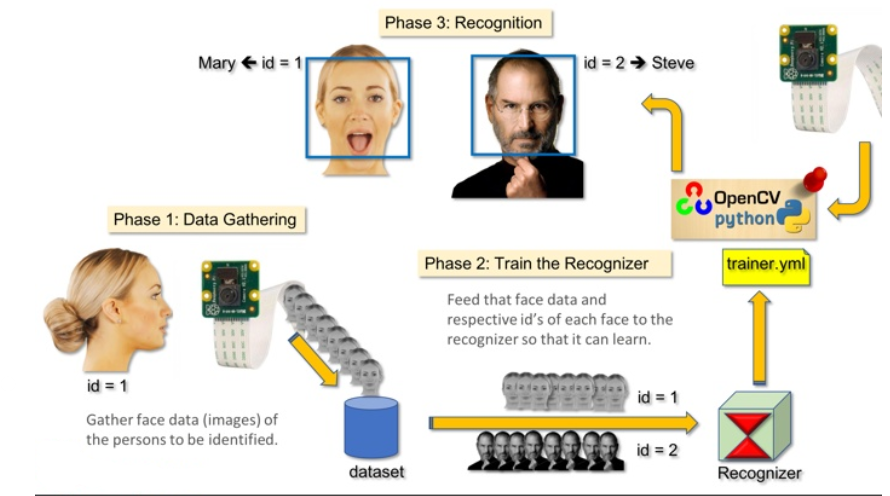#### 第2步：安装OpenCV 包

sudo apt-get install libopencv-dev
sudo apt-get install python-opencv


import cv2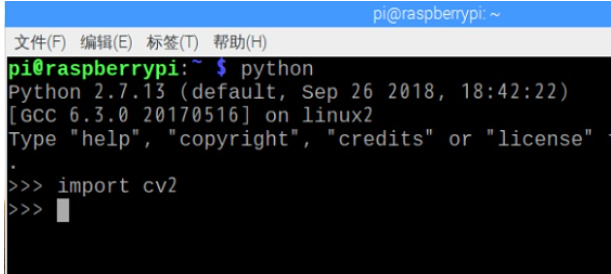cv2.__version__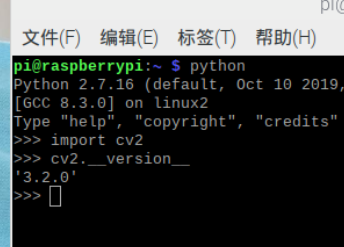#### 1.硬件安装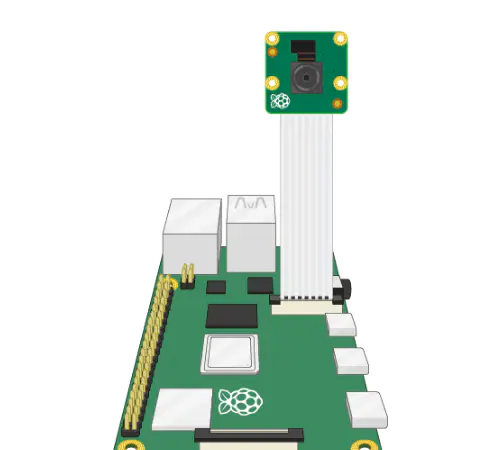#### 2.打开Raspberry Pi配置工具开启Camera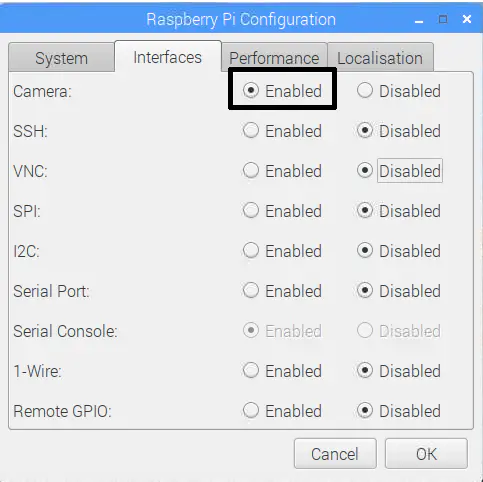vcgencmd get_camera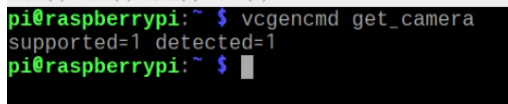raspistill -o image.jpg


testopencv.py

git clone https://ghp.quickso.cn/https://raw.githubusercontent.com/TommyZihao/opencvtest/master/testopencv.py
cd opencvtest
python2 testopencv.py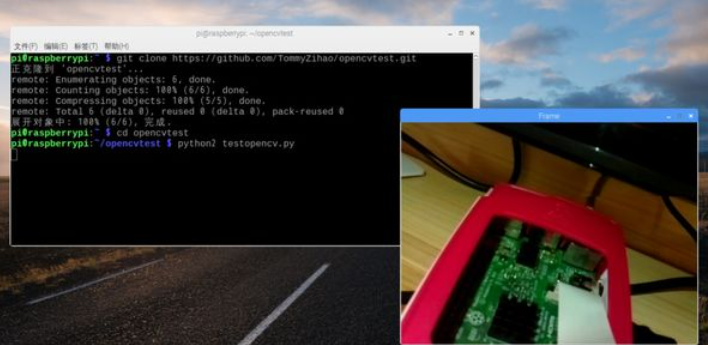#### 第4步：人脸检测

import numpy as np
import cv2

cap = cv2.VideoCapture(0)
cap.set(3,640) # set Width
cap.set(4,480) # set Height

while True:
img = cv2.flip(img, 1)
gray = cv2.cvtColor(img, cv2.COLOR_BGR2GRAY)
gray,

scaleFactor=1.2,
minNeighbors=5
,
minSize=(20, 20)
)

for (x,y,w,h) in faces:
cv2.rectangle(img,(x,y),(x+w,y+h),(255,0,0),2)
roi_gray = gray[y:y+h, x:x+w]
roi_color = img[y:y+h, x:x+w]

cv2.imshow('video',img)

k = cv2.waitKey(30) & 0xff
if k == 27: # press 'ESC' to quit
break

cap.release()
cv2.destroyAllWindows()


faceCascade = cv2.CascadeClassifier('haarcascade_frontalface_default.xml')


faces = faceCascade.detectMultiScale(
gray,
scaleFactor=1.2,
minNeighbors=5,
minSize=(20, 20)
)


gray 表示输入 grayscale 图像。
scaleFactor 表示每个图像缩减的比例大小。
minNeighbors 表示每个备选矩形框具备的邻近数量。数字越大，假正类越少。
minSize 表示人脸识别的最小矩形大小。

for (x,y,w,h) in faces:
cv2.rectangle(img,(x,y),(x+w,y+h),(255,0,0),2)
roi_gray = gray[y:y+h, x:x+w]
roi_color = img[y:y+h, x:x+w]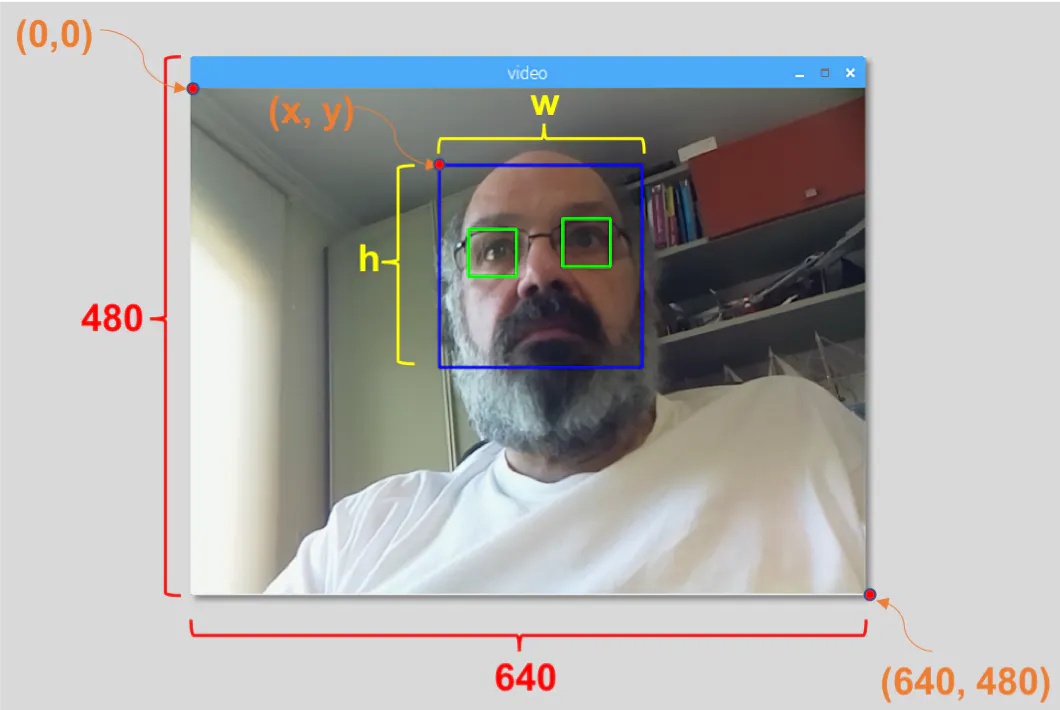python faceDetection.py


faceEyeDetection.py
faceSmileDetection.py
faceSmileEyeDetection.py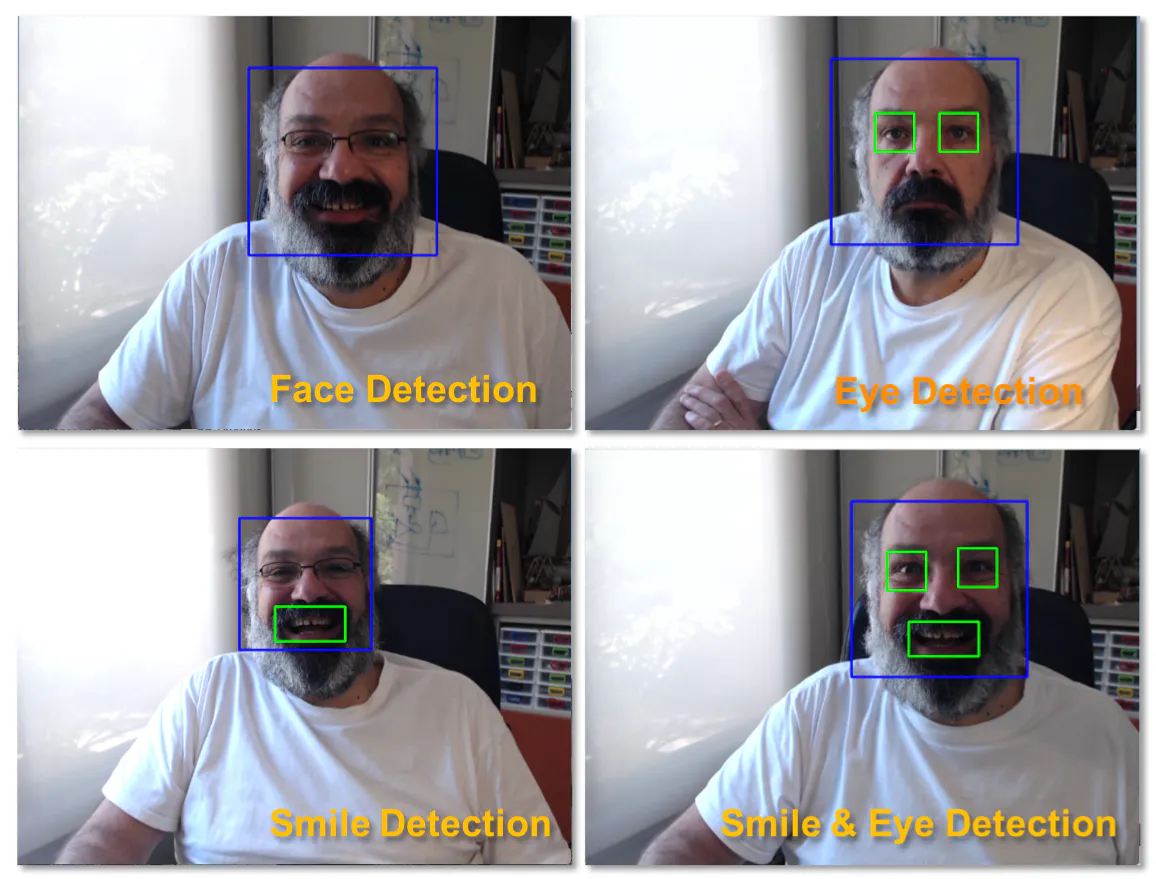#### 第5步：收集数据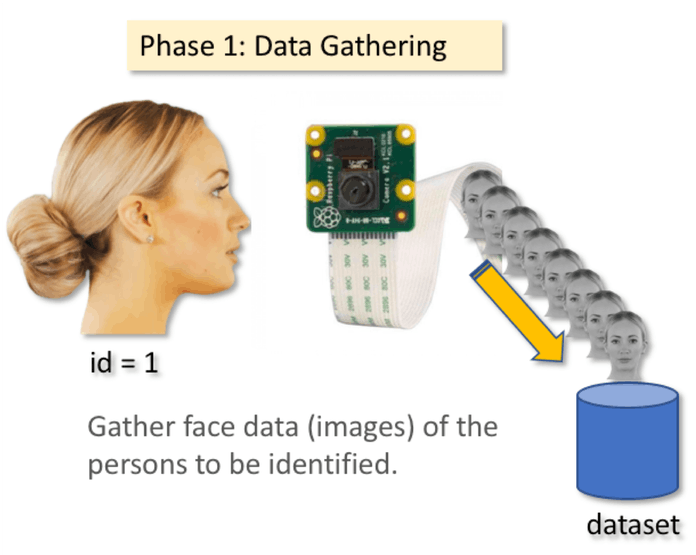mkdir FacialRecognitionProject


mkdir dataset


import cv2
import os

cam = cv2.VideoCapture(0)
cam.set(3, 640) # set video width
cam.set(4, 480) # set video height

# For each person, enter one numeric face id
face_id = input('\n enter user id end press <return> ==>  ')

print("\n [INFO] Initializing face capture. Look the camera and wait ...")
# Initialize individual sampling face count
count = 0

while(True):

img = cv2.flip(img, 1) # flip video image vertically
gray = cv2.cvtColor(img, cv2.COLOR_BGR2GRAY)
faces = face_detector.detectMultiScale(gray, 1.3, 5)

for (x,y,w,h) in faces:

cv2.rectangle(img, (x,y), (x+w,y+h), (255,0,0), 2)
count += 1

# Save the captured image into the datasets folder
cv2.imwrite("dataset/User." + str(face_id) + '.' + str(count) + ".jpg", gray[y:y+h,x:x+w])

cv2.imshow('image', img)

k = cv2.waitKey(100) & 0xff # Press 'ESC' for exiting video
if k == 27:
break
elif count >= 30: # Take 30 face sample and stop video
break

# Do a bit of cleanup
print("\n [INFO] Exiting Program and cleanup stuff")
cam.release()
cv2.destroyAllWindows()



face_id = input('\n enter user id end press  ==>  ')


cv2.imwrite("dataset/User." + str(face_id) + '.' + str(count) + ".jpg", gray[y:y+h,x:x+w])


User.face_id.count.jpg


User.1.4.jpg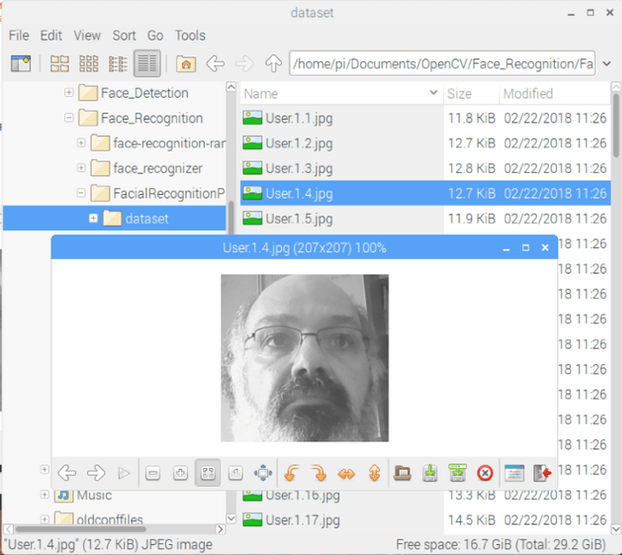#### 第六步：训练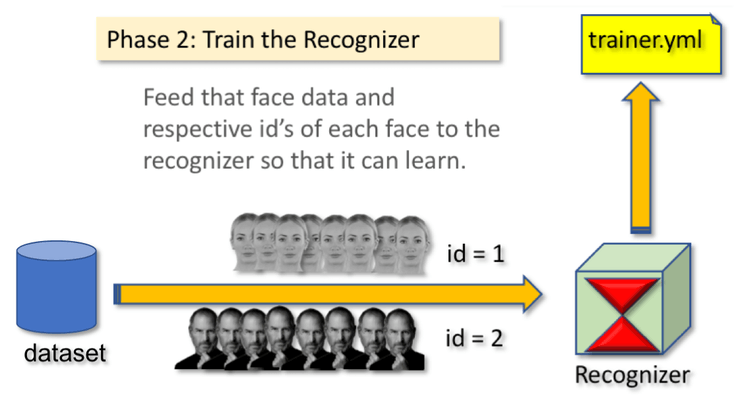mkdir trainer


import cv2
import numpy as np
from PIL import Image
import os

# Path for face image database
path = 'dataset'

recognizer = cv2.face.createLBPHFaceRecognizer()

# function to get the images and label data
def getImagesAndLabels(path):

imagePaths = [os.path.join(path,f) for f in os.listdir(path)]
faceSamples=[]
ids = []

for imagePath in imagePaths:

PIL_img = Image.open(imagePath).convert('L') # convert it to grayscale
img_numpy = np.array(PIL_img,'uint8')

id = int(os.path.split(imagePath)[-1].split("."))
faces = detector.detectMultiScale(img_numpy)

for (x,y,w,h) in faces:
faceSamples.append(img_numpy[y:y+h,x:x+w])
ids.append(id)

return faceSamples,ids

print ("\n [INFO] Training faces. It will take a few seconds. Wait ...")
faces,ids = getImagesAndLabels(path)
recognizer.train(faces, np.array(ids))

# Save the model into trainer/trainer.yml
recognizer.save('trainer/trainer.yml')

# Print the numer of faces trained and end program
print("\n [INFO] {0} faces trained. Exiting Program".format(len(np.unique(ids))))


1.在python3中，cv2.face.createLBPHFaceRecognizer()方法已经修改成为：
recognizer = cv2.face.LBPHFaceRecognizer_create()
2.recognizer.write('trainer/trainer.yml')  #Python3中用这个
recognizer.save('trainer/trainer.yml') #Python2中用这个


pip install pillow


recognizer = cv2.face.createLBPHFaceRecognizer()


recognizer.train(faces, ids)


#### 第7步：识别器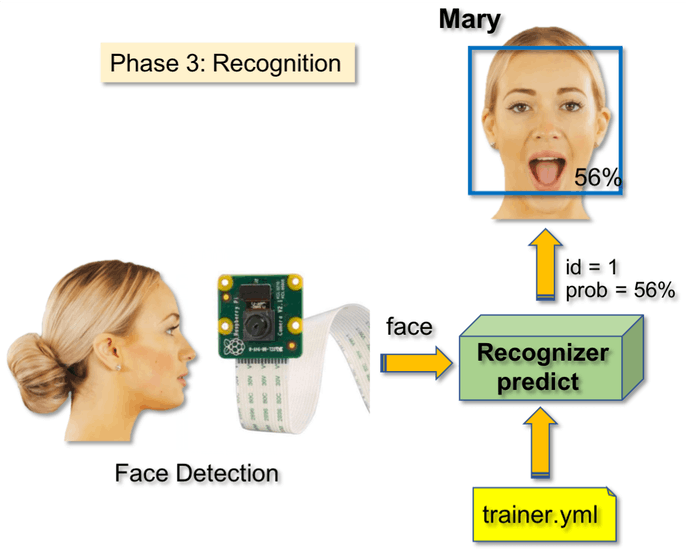import cv2
import numpy as np
import os

recognizer = cv2.face.createLBPHFaceRecognizer()

font = cv2.FONT_HERSHEY_SIMPLEX

#iniciate id counter
id = 0

# names related to ids: example ==> Marcelo: id=1,  etc
names = ['None','Marcelo', 'Paula', 'Ilza', 'Z', 'W']

# Initialize and start realtime video capture
cam = cv2.VideoCapture(0)
cam.set(3, 640) # set video widht
cam.set(4, 480) # set video height

# Define min window size to be recognized as a face
minW = 0.1*cam.get(3)
minH = 0.1*cam.get(4)

while True:

img = cv2.flip(img, 1) # Flip vertically

gray = cv2.cvtColor(img,cv2.COLOR_BGR2GRAY)

gray,
scaleFactor = 1.2,
minNeighbors = 5,
minSize = (int(minW), int(minH)),
)

for(x,y,w,h) in faces:

cv2.rectangle(img, (x,y), (x+w,y+h), (0,255,0), 2)

id, confidence = recognizer.predict(gray[y:y+h,x:x+w])

# Check if confidence is less them 100 ==> "0" is perfect match
if (confidence < 100):
id = names[id]
confidence = "  {0}%".format(round(100 - confidence))
else:
id = "unknown"
confidence = "  {0}%".format(round(100 - confidence))

cv2.putText(img, str(id), (x+5,y-5), font, 1, (255,255,255), 2)
cv2.putText(img, str(confidence), (x+5,y+h-5), font, 1, (255,255,0), 1)

cv2.imshow('camera',img)

k = cv2.waitKey(10) & 0xff # Press 'ESC' for exiting video
if k == 27:
break

# Do a bit of cleanup
print("\n [INFO] Exiting Program and cleanup stuff")
cam.release()
cv2.destroyAllWindows()


recognizer.load('trainer/trainer.yml') #Python2用这个


names = ['None', 'Marcelo', 'Paula', 'Ilza', 'Z', 'W']


id, confidence = recognizer.predict(gray portion of the face)


recognizer.predict () 将把待分析人脸的已捕捉部分作为一个参数，并返回其可能的所有者，指示其 id 以及识别器与这一匹配相关的置信度。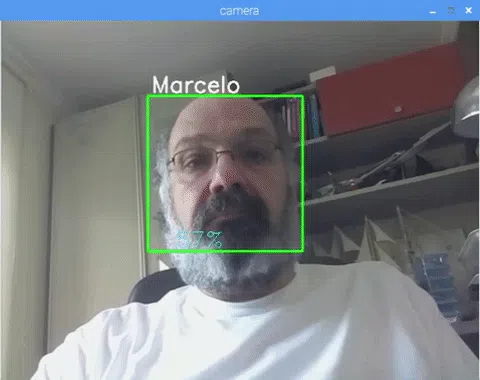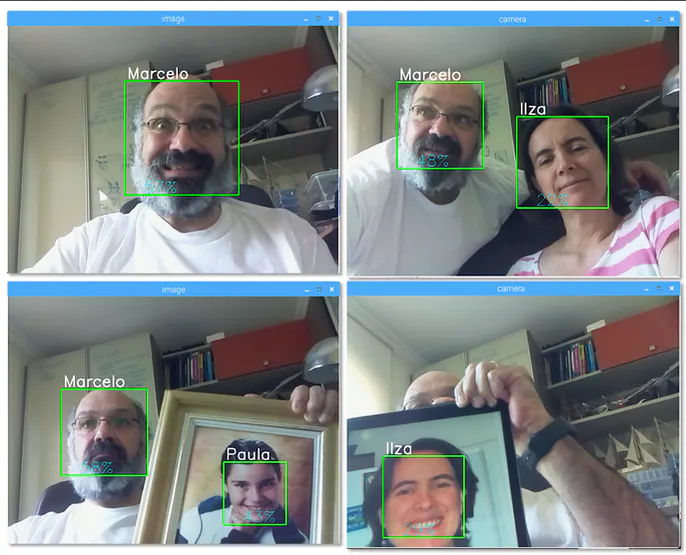#### 第 8 步：结语

muzihuaner/Raspbian_Opencv_Python2_Facerecognition: 在树莓派上基于Python2进行人脸识别 (github.com)

posted @ 2021-10-18 19:36  木子欢儿  阅读(238)  评论(0编辑  收藏  举报